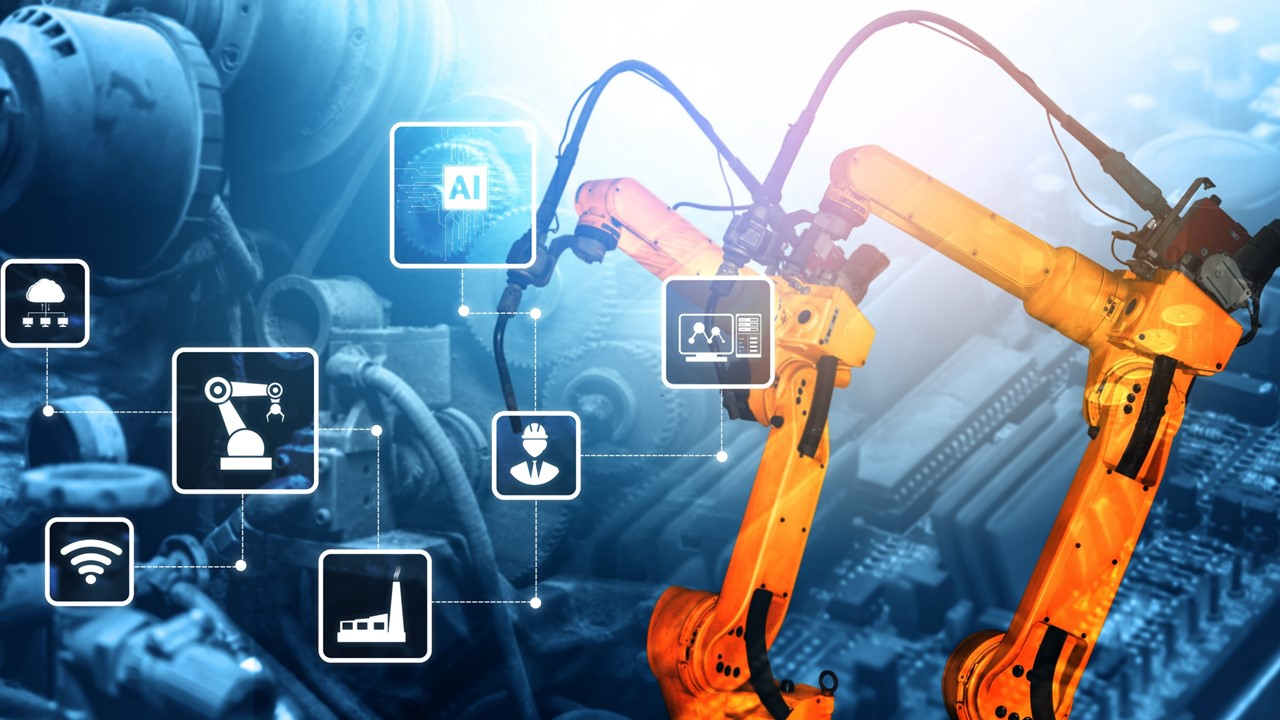# Understanding Variational Auto-encoders

Variational Auto-encoders (VAEs) are a type of generative model that combines the concepts of auto-encoders and variational inference. Autoencoders are neural network architectures used for unsupervised learning, which aim to encode high-dimensional input data into a lower-dimensional latent space and then decode it back to reconstruct the original input. Variational inference, on the other hand, is a statistical technique used to approximate complex probability distributions.

The main idea behind VAEs is to train an auto-encoder to learn a latent representation that not only captures the salient features of the input data but also follows a specific probability distribution, typically a Gaussian distribution. This property enables VAEs to generate new samples by sampling from the learned latent space.

The architecture of a VAE consists of two main components: an encoder and a decoder. The encoder takes the input data and maps it to a latent space distribution. Instead of directly outputting the latent variables, the encoder produces two vectors: the mean vector (μ) and the standard deviation vector (σ). These vectors define the parameters of the approximate latent distribution.Once the encoder has produced the mean and standard deviation vectors, the sampling process takes place. Random samples are drawn from a standard Gaussian distribution, which are then multiplied by the standard deviation vector (σ) and added to the mean vector (μ) to obtain the latent variables (z). These latent variables are the input to the decoder.

The decoder takes the latent variables and attempts to reconstruct the original input data. It maps the latent space back to the input space and produces a reconstructed output. The reconstruction is optimized to be as close as possible to the original input using a loss function, typically the mean squared error or binary cross-entropy loss.

During training, VAEs aim to optimize two objectives simultaneously: reconstruction loss and regularization loss. The reconstruction loss measures the discrepancy between the input and the reconstructed output, encouraging the model to capture the important features of the data. The regularization loss, also known as the Kullback-Leibler (KL) divergence, enforces the learned latent distribution to match a desired prior distribution (often a standard Gaussian distribution). This encourages the latent space to be well-structured and smooth.

Once a VAE is trained, it can generate new samples by sampling from the learned latent space. By providing random samples from the prior distribution and passing them through the decoder, the VAE can produce new data points that resemble the training data.

Variational Auto-encoders have gained popularity for their ability to learn meaningful latent representations and generate novel data. They have been successfully applied to tasks such as image generation, data compression, anomaly detection, and semi-supervised learning.

##### Related Articles##### Influence of Enterprise environmental factors in project management##### Are Design Thinking and Six Sigma mutually exclusive?##### The pros and cons between classic machine learning methods and deep learning